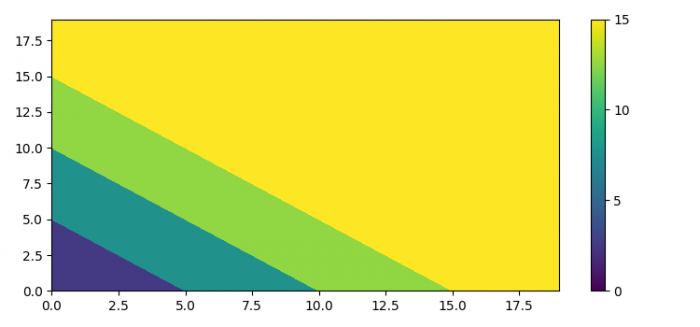# Setting the limits on a colorbar of a contour plot in Matplotlib

To set the limits on a colorbar of a countour plot in Matplotlib, we can take the following steps −

• Set the figure size and adjust the padding between and around the subplots.
• Create x and y data points using numpy.
• Get the data using x and y.
• Get the coordinate matrices from the coordinate vectors.
• Initialize vmin and vmax to set the limits on a colorbar of a contour plot in matplotlib.
• Plot contours using contourf() method.
• Make the colorbar using scalar mappable within the range of vmin and vmax.
• To display the figure, use show() method.

## Example

from matplotlib import pyplot as plt
import numpy as np
from matplotlib.cm import ScalarMappable

plt.rcParams["figure.figsize"] = [7.50, 3.50]
plt.rcParams["figure.autolayout"] = True

x = np.arange(20)
y = np.arange(20)

data = x[:, None] + y[None, :]
X, Y = np.meshgrid(x, y)

vmin = 0
vmax = 15

fig, ax = plt.subplots()

qcs = ax.contourf(
X, Y, data,
vmin=vmin, vmax=vmax
)

fig.colorbar(
ScalarMappable(norm=qcs.norm, cmap=qcs.cmap),
ticks=range(vmin, vmax+5, 5)
)

plt.show()

## Output